# Interaction Overview Diagram

## How To draw UML Interaction Overview Diagrams

UML Interaction Overview Diagram schematically shows a control flow with nodes and a sequence of activities that can contain interaction or sequence diagrams.

ConceptDraw has examples that help you to start using software for drawing UML Diagrams. You can use the appropriate stencils of UML notation from UML Interaction Overview library with 13 objects.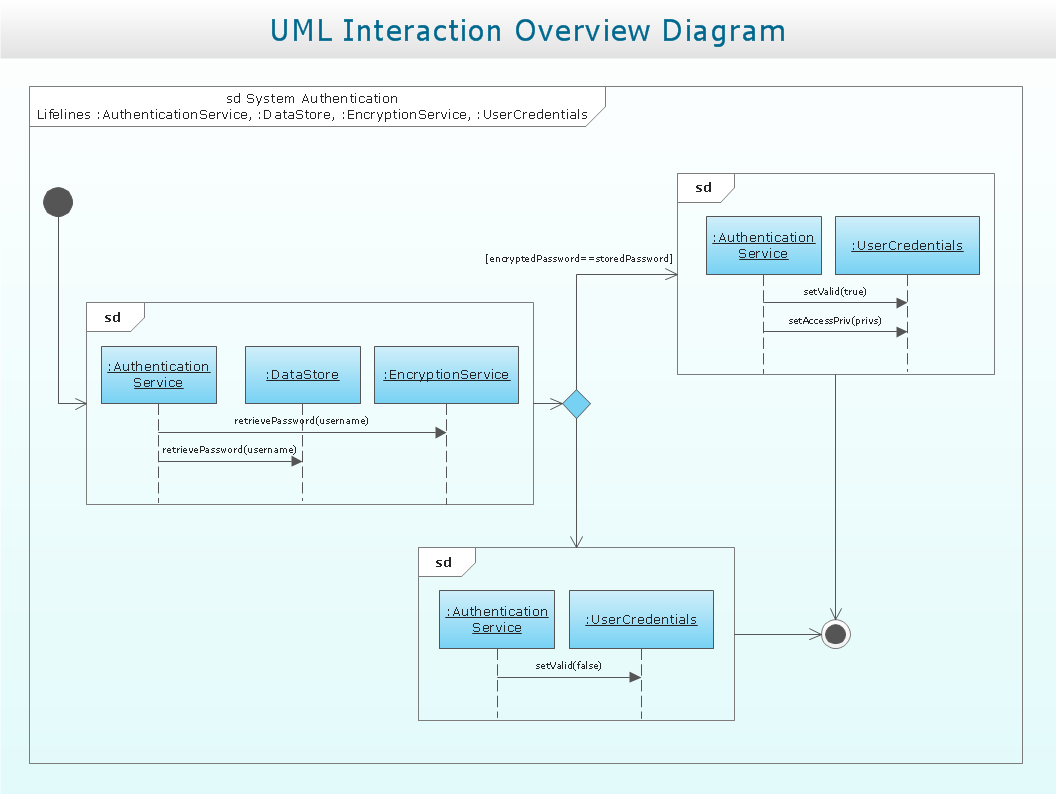Sample 1. UML Interaction Overview Diagram. System authentication

This example is created using ConceptDraw DIAGRAM diagramming and vector drawing software enhanced with Rapid UML solution from ConceptDraw Solution Park.

Rapid UML solution provides templates, examples and libraries of stencils for quick and easy drawing UML 2.4 interaction overview diagram.

Use ConceptDraw DIAGRAM with UML Interaction Overview Diagram templates, samples and stencil library from Rapid UML solution to model a control flow of your system with nodes that can contain interaction or sequence diagrams.

### UML Component Diagram Example - Online Shopping

UML Component Diagram Online Shopping. This sample was created in ConceptDraw DIAGRAM v12 diagramming and vector drawing software using the UML Component Diagram library of the Rapid UML Solution from the Software Development area of ConceptDraw Solution Park. This sample shows the concept of the online shopping and is used for the understanding of the online shopping processes, of the online shops working processes, for projection and creating of the online stores.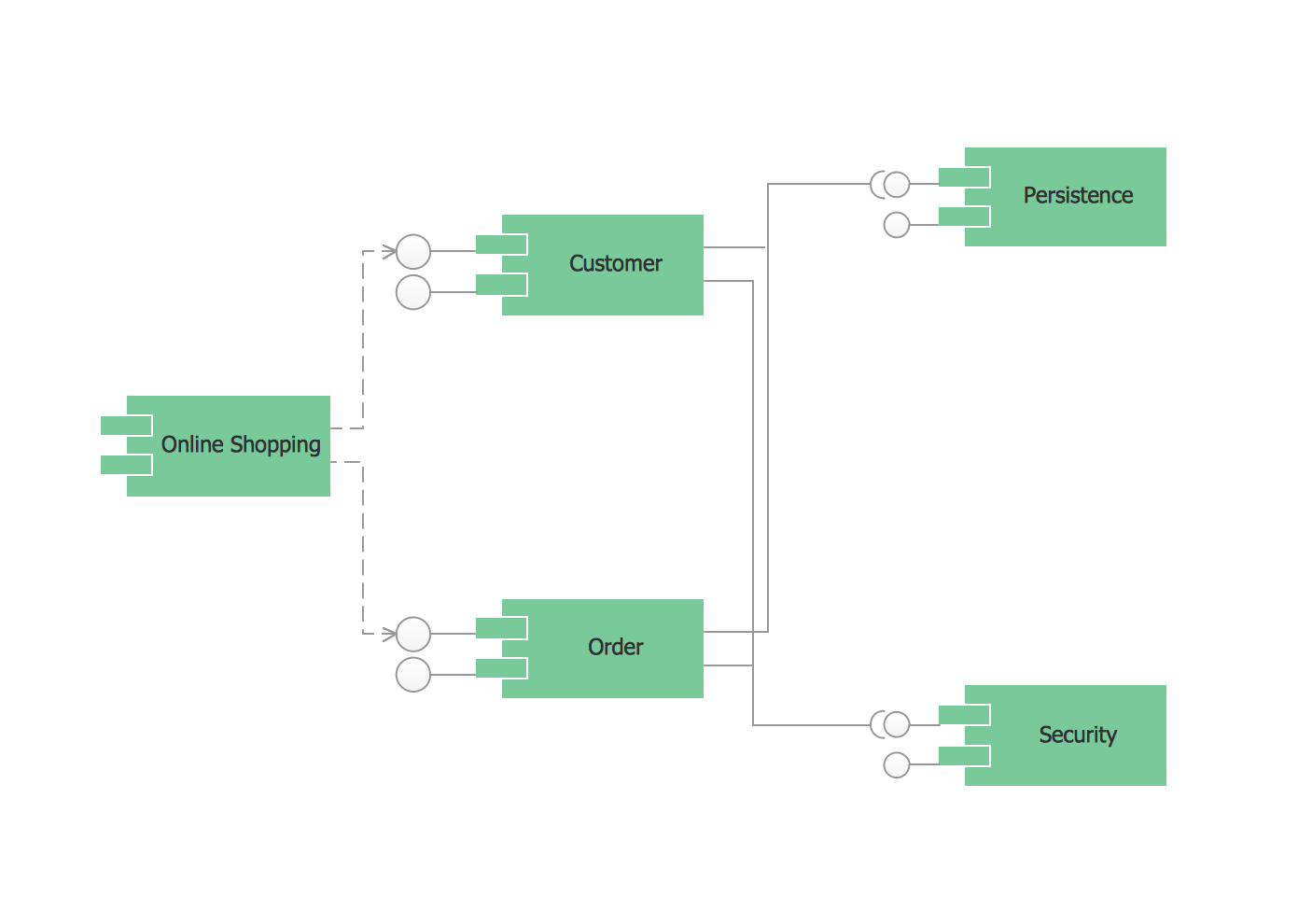Picture: UML Component Diagram Example - Online Shopping
Related Solution:

### Data Flow Diagram Symbols. DFD Library

Any information system receives data flows from external sources. In order to visualize them there is a list of data flow diagram symbols that describes how the system components cooperate. If you want to create a data flow diagram, ConceptDraw DIAGRAM v12 Solution Park has DFD Library that contains both Yourdon and Gane-Sarson notations. This figure shows the content of vector libraries, delivered with ConceptDraw solution for data flow diagram (DFD). There are three libraries composed from about 50 vector objects used to make data flow diagrams. They include a complete set of objects utilized by Yourdon-Coad and Gane-Sarson notations - two primary notations that are apply for data flow diagramming. Also, one can discover additional "Data flow diagram (DFD)" library that provides a data flow diagram elements for designing level 1 and context-level data flow diagrams.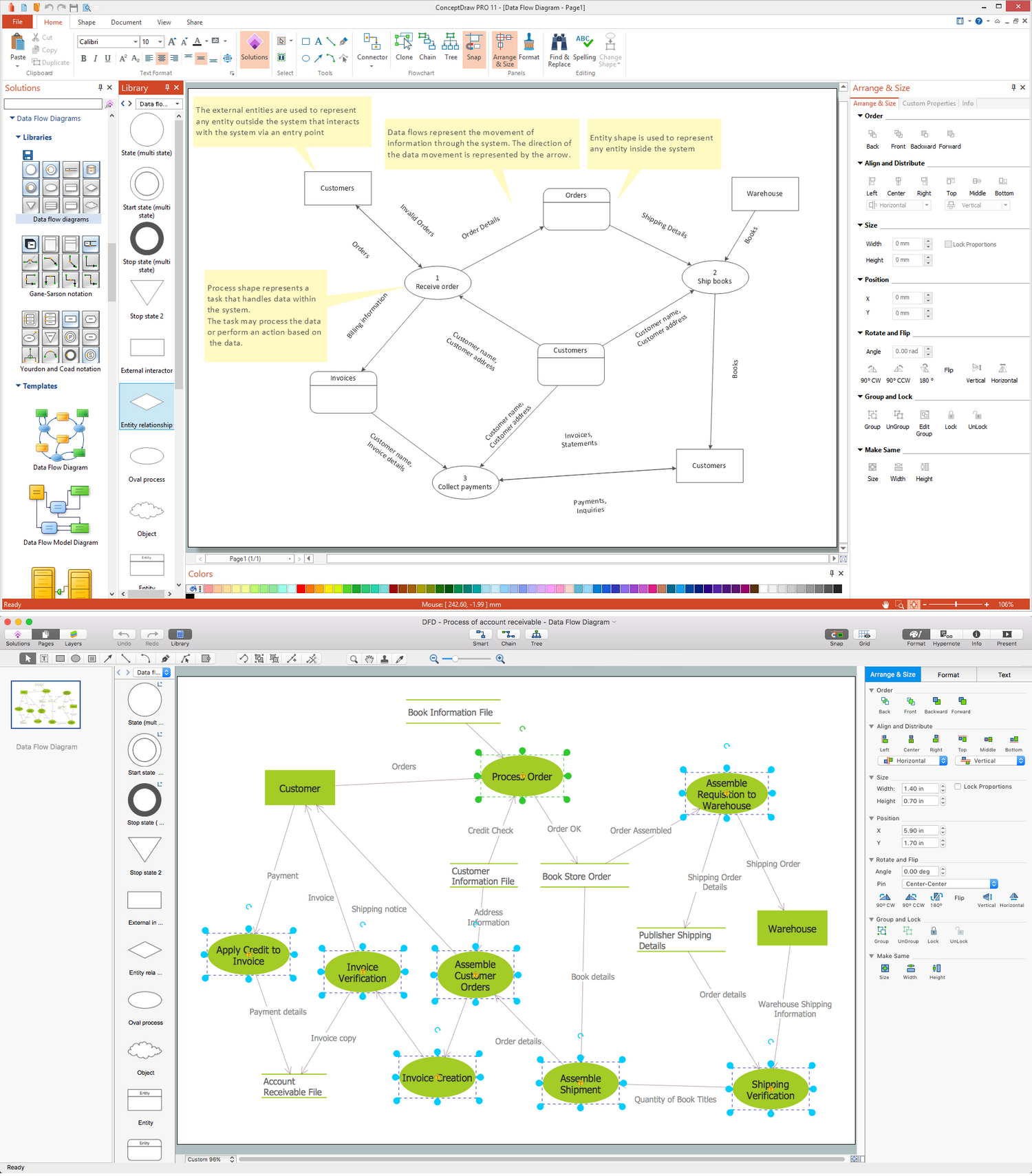Picture: Data Flow Diagram Symbols. DFD Library
Related Solution:

### UML Diagram

While developing software, it is very important to have a visual model, because it helps to represent the logic and the architecture of an application. Experienced engineers use UML diagrams to denote relationships between classes and their instances. UML is a general language for a set of diagrams like deployment diagrams, object diagrams or use case diagrams. This diagram represents UML class diagram used for a software system development using an object-oriented method. Class diagrams are categorized as static structure diagrams that depict the physical structure of a system. Class diagram divides a software system's structure into "classes". Classes are defined by the methods and variables of objects. UML Class diagram is used to depict relationships and source code dependencies between objects.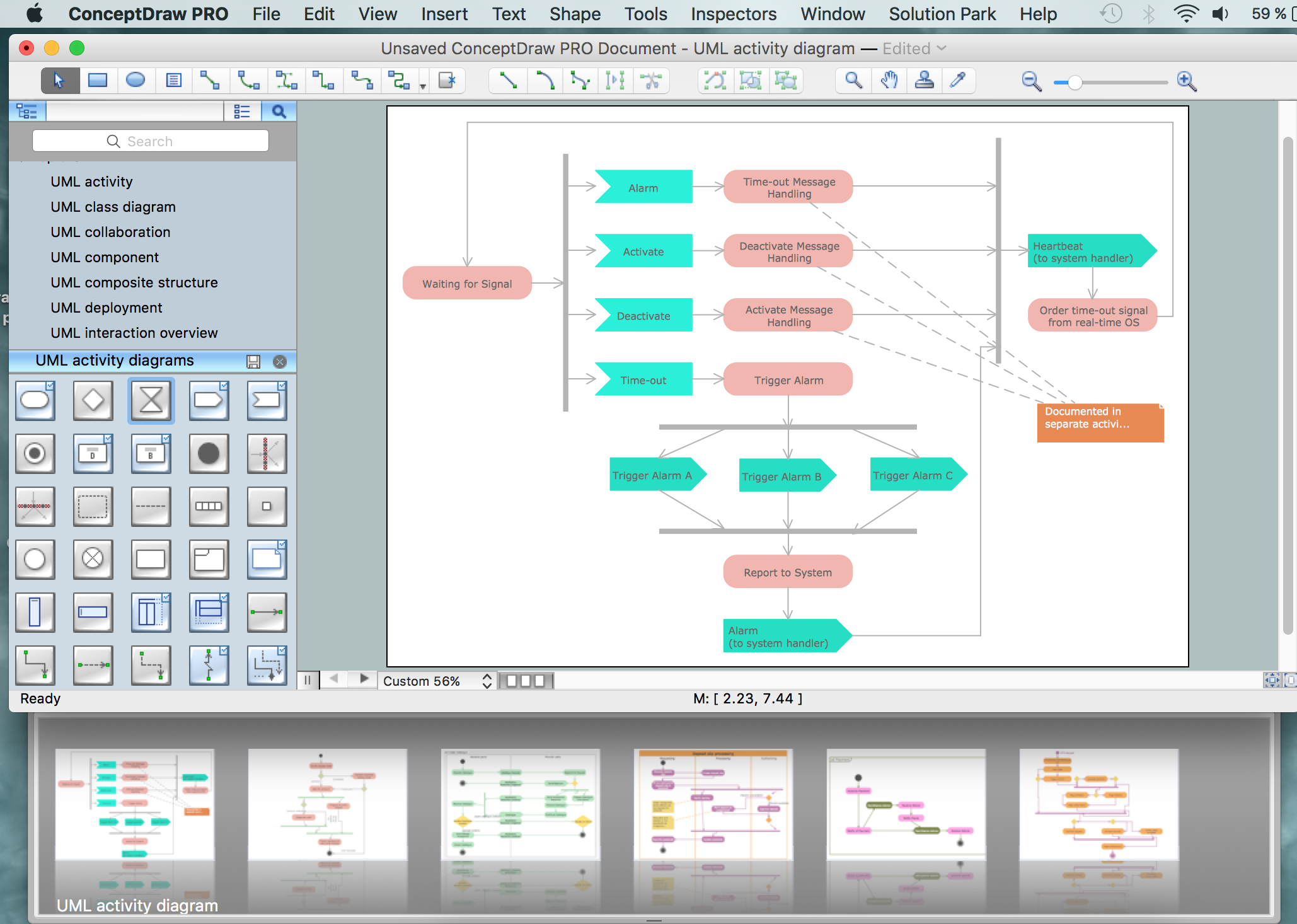Picture: UML Diagram
Related Solution:

### UML Use Case Diagram Example Registration System

This sample was created in ConceptDraw DIAGRAM v12 diagramming and vector drawing software using the UML Use Case Diagram library of the Rapid UML Solution from the Software Development area of ConceptDraw Solution Park. This sample shows the types of userâ€™s interactions with the system and is used at the registration and working with the database system.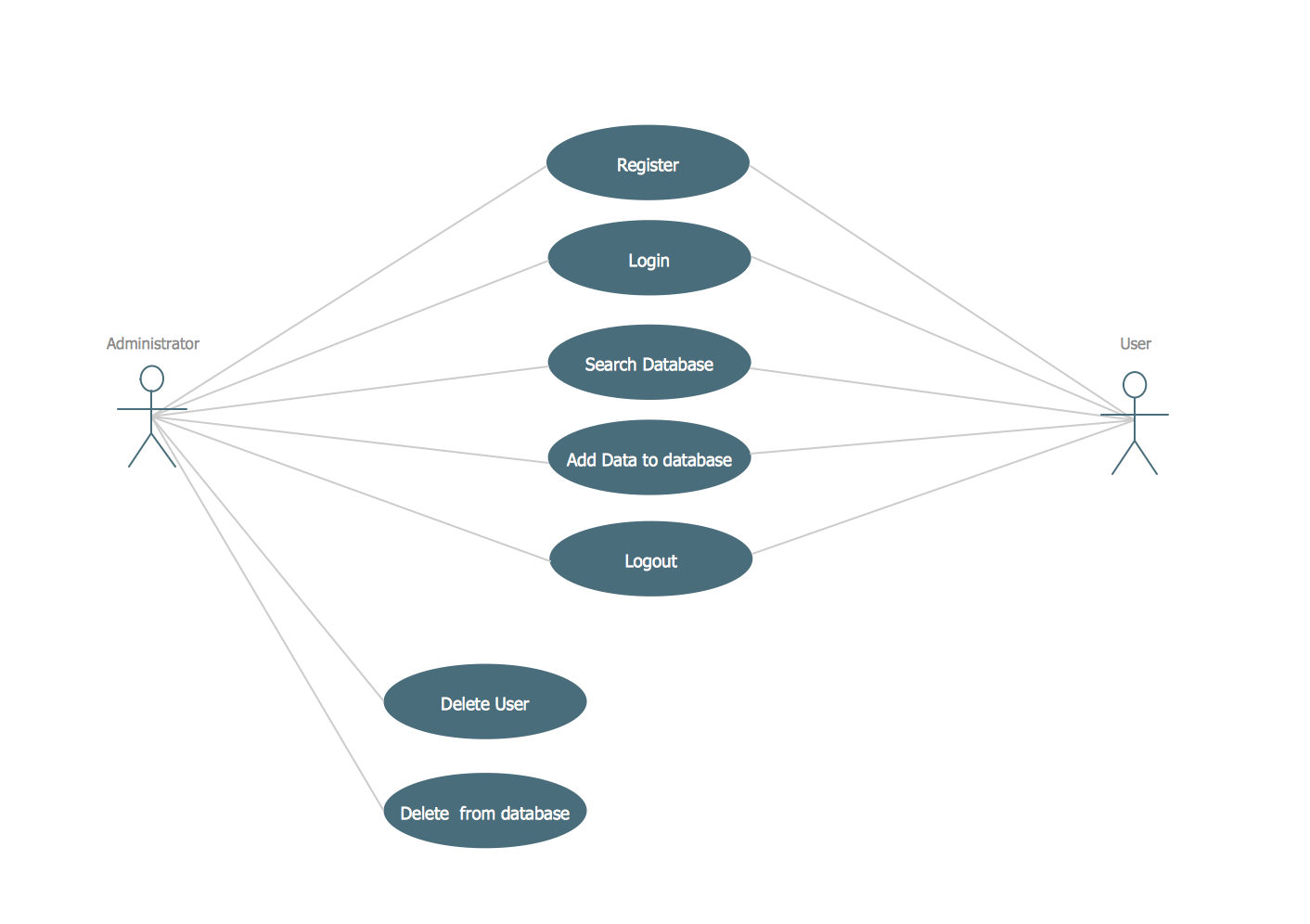Picture: UML Use Case Diagram Example Registration System
Related Solution:

### Diagramming Software for Design UML Use Case Diagrams

Use Case Diagrams describes the functionality provided by a system in terms of actors, their goals represented as use cases, and any dependencies among those use cases.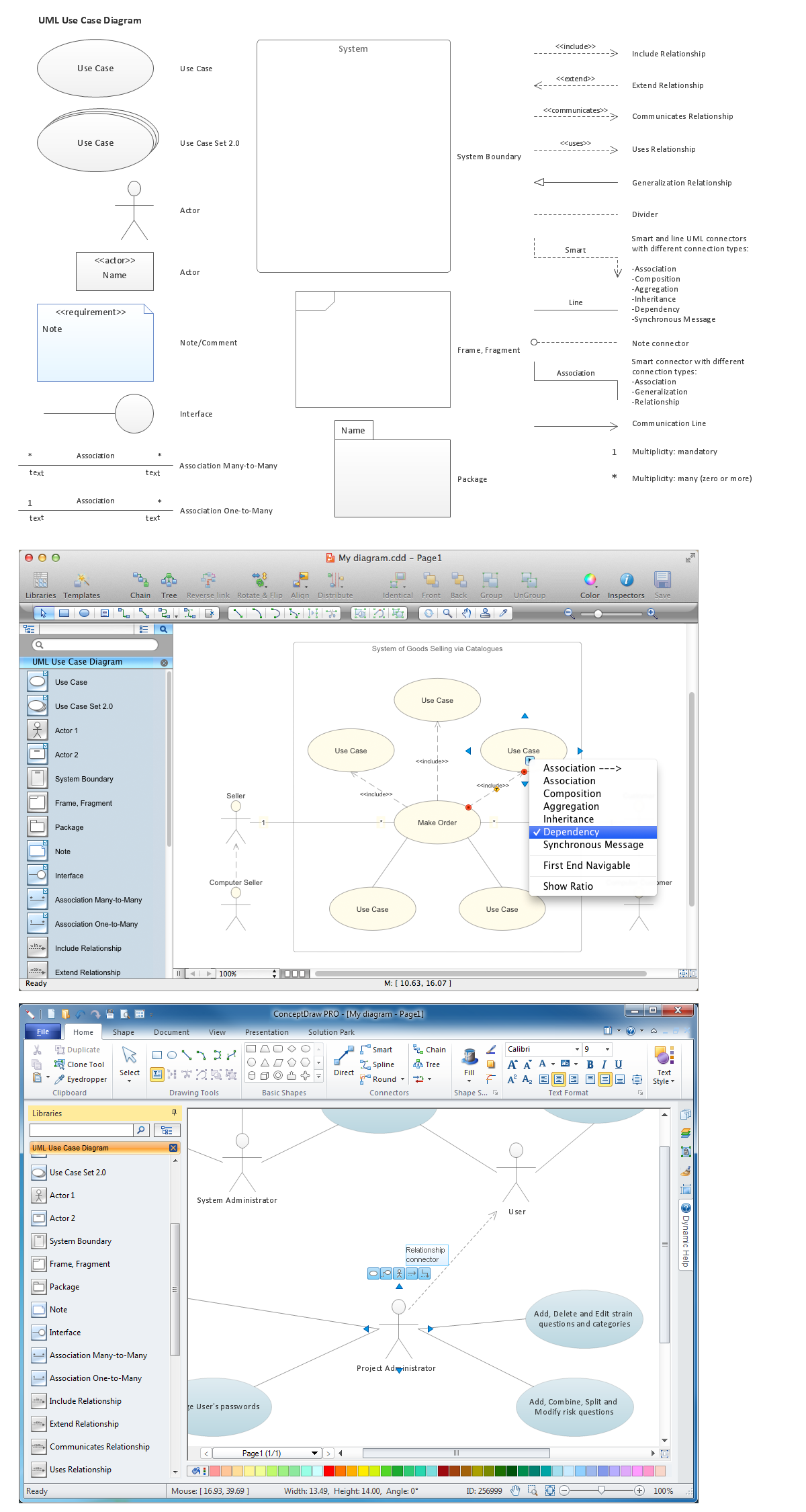Picture: Diagramming Software for Design UML Use Case Diagrams

### Diagramming Software for Design UML Collaboration Diagrams

ConceptDraw helps you to start designing your own UML Collaboration Diagrams with examples and templates.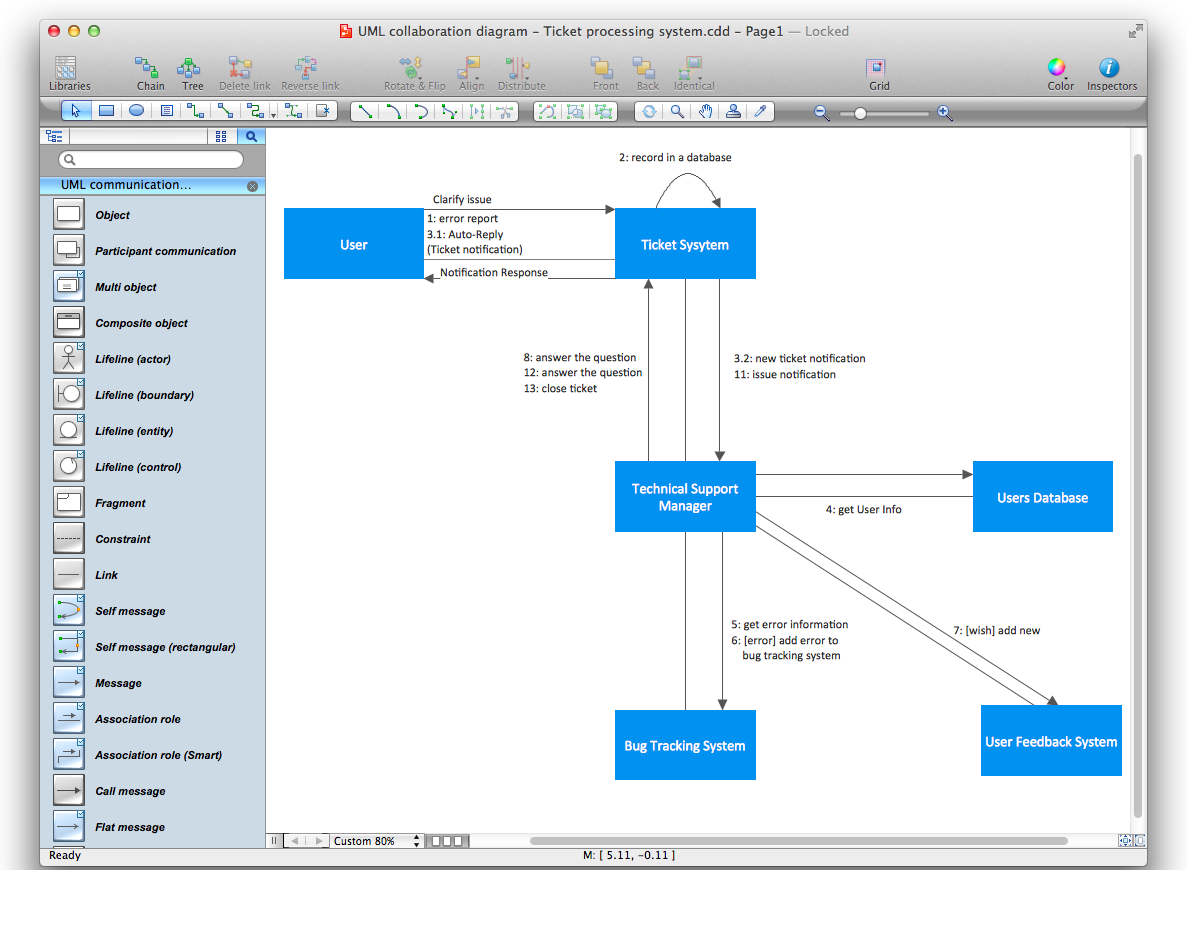Picture: Diagramming Software for Design UML Collaboration Diagrams

### Diagramming Software for Design UML Interaction Overview Diagrams

UML Interaction Overview Diagram schematically shows a control flow with nodes and a sequence of activities that can contain interaction or sequence diagrams.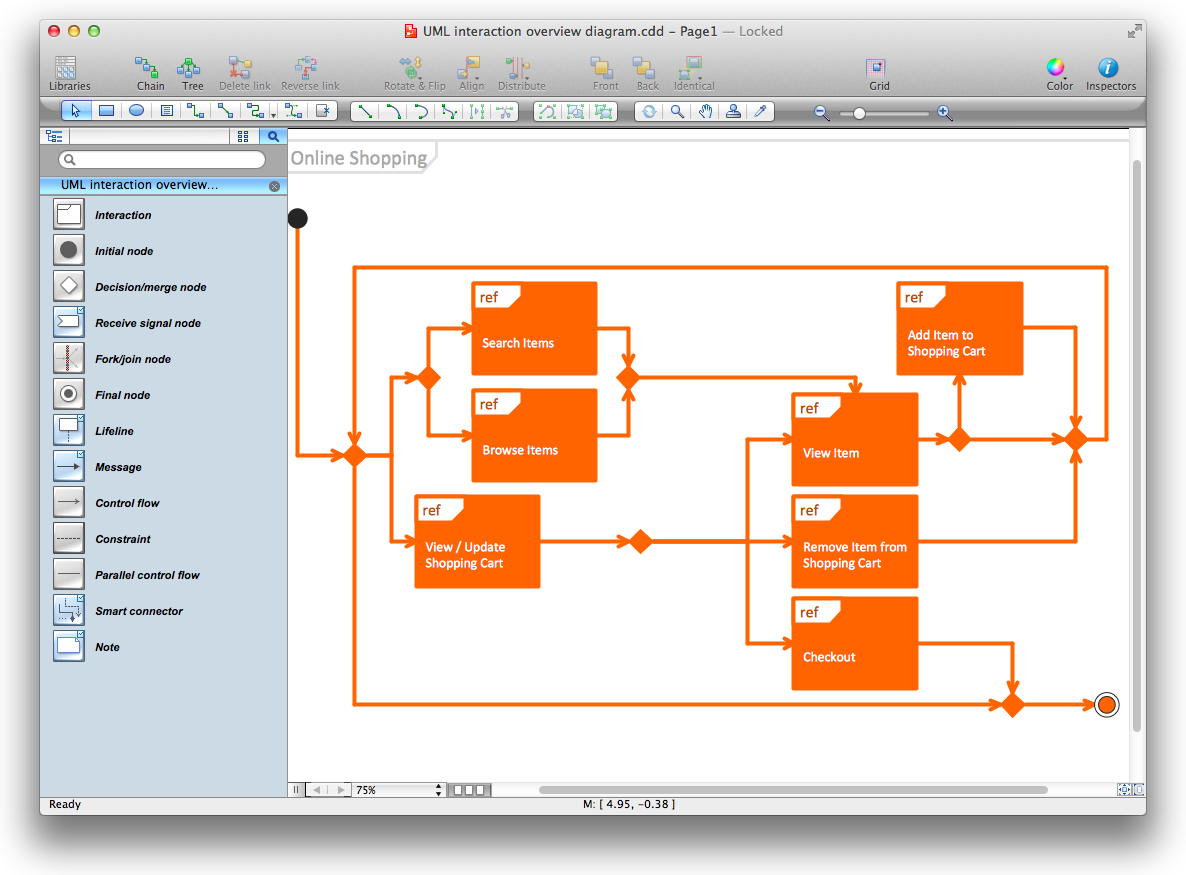Picture: Diagramming Software for Design UML Interaction Overview Diagrams

### UML Component Diagram

UML Component Diagrams are used to illustrate the structure of arbitrarily complex systems and illustrates the service consumer - service provider relationship between components.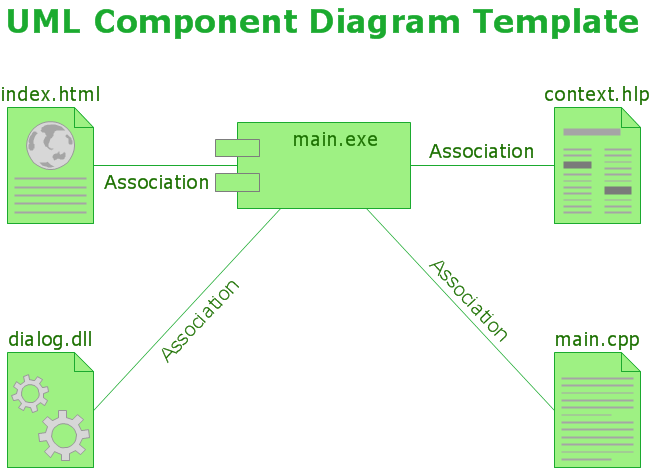Picture: UML Component Diagram

### UML Component Diagram. Design Elements

UML Component Diagram illustrates show components are wired together to larger components and software systems that shows the structure of arbitrarily complex systems. Rapid UML Solution for ConceptDraw DIAGRAM v12 contains 13 vector stencils libraries with 393 interactive shapes that you can use to design your UML diagrams. To design a Component Diagram use the UML Component Diagram library. UML Component Diagram library contains 36 shapes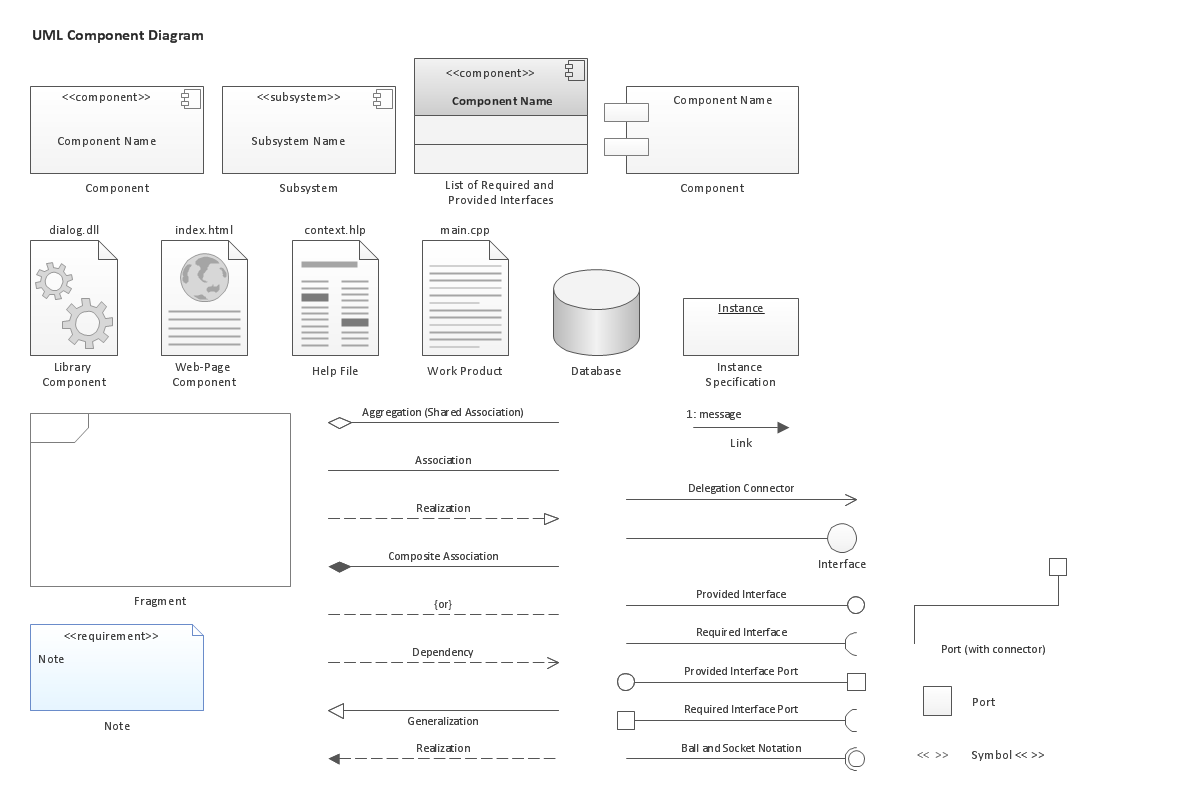Picture: UML Component Diagram. Design Elements
Related Solution:

### UML Deployment Diagram Example - ATM SystemUML diagrams

This sample was created in ConceptDraw DIAGRAM v12 diagramming and vector drawing software using the UML Deployment Diagram library of the Rapid UML Solution from the Software Development area of ConceptDraw Solution Park. This sample shows the work of the ATM (Automated Teller Machine) banking system that is used for service and performing of the banking transactions using ATMs. System engineers can use comprehensive UML diagrams solution.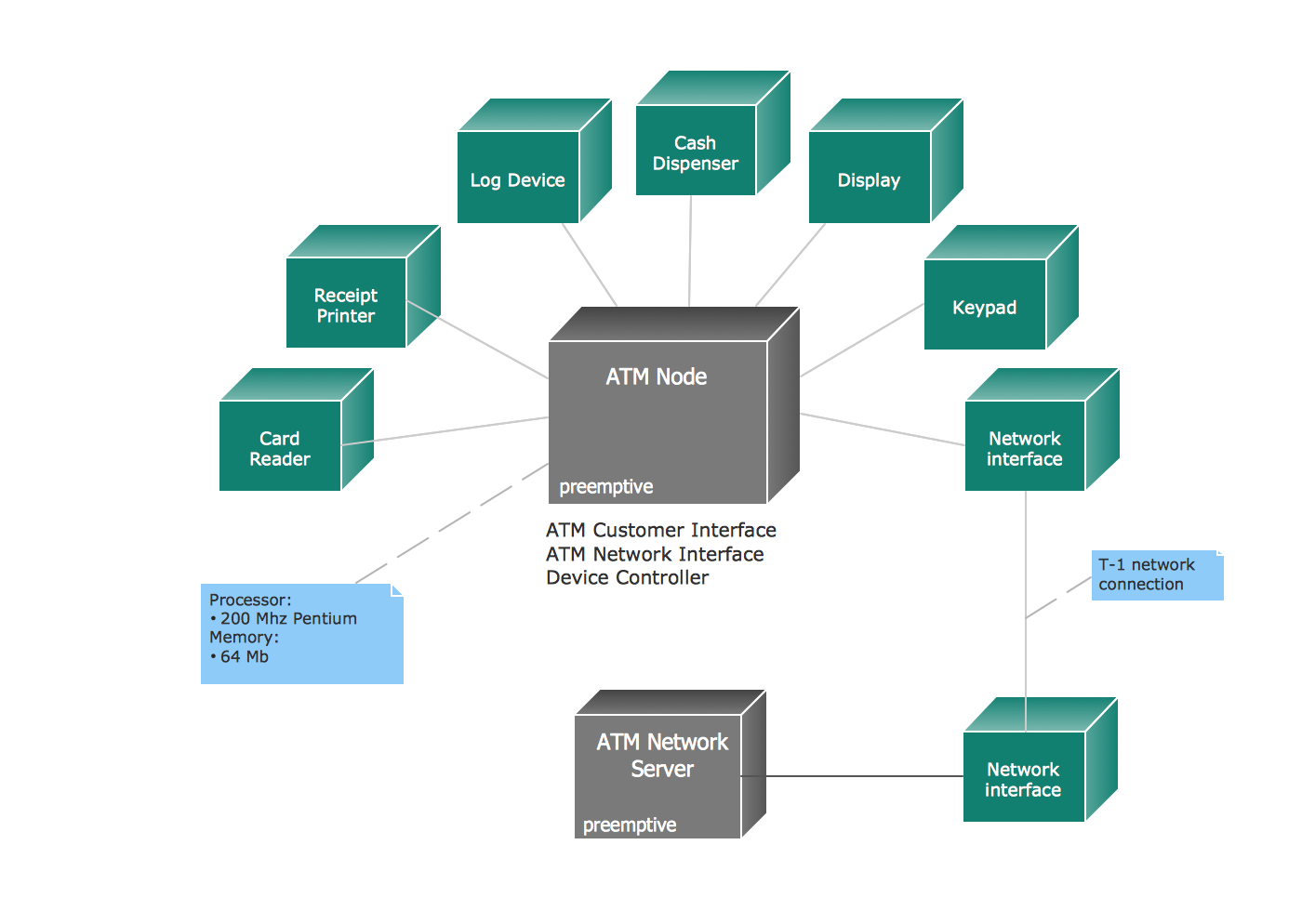Picture: UML Deployment Diagram Example - ATM SystemUML diagrams
Related Solution: